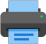Lista de exercícios do ensino médio para impressãoSelect all.
print selected exercises
The circle as shown in the picture below, with center P and radius 2, is tangent to three sides of the rectangle ABCD. Given that the total area of the rectangle is 32, find the distance between the point P and the diagonal AC.
a)
$\,2\dfrac{\sqrt{5}}{5}\,$
b)
$\,\dfrac{\sqrt{5}}{2}\,$
c)
$\,\dfrac{\sqrt{5}}{5}\,$
d)
$\,2\sqrt{5}\,$
e)
$\,3\dfrac{\sqrt{5}}{5}\,$